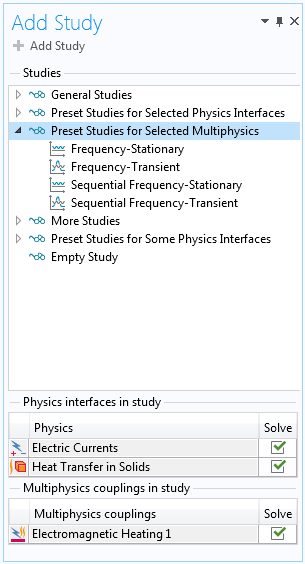# Solving electromagnetic heating models with high-frequency loads

 解决方法编号: 1257 标题: Solving electromagnetic heating models with high-frequency loads 平台: 类别: General, Solver 关键字:

## 问题描述

I am solving a Multiphysics problem involving electromagnetics and heat transfer. The electromagnetic fields are varying at a constant frequency, and the period is very short compared to the timespan over which the system heats up. In addition, the electrical properties are a function of the changing temperature over time. How should I set up such problems efficiently?

## 解决方法

### Background

Many Multiphysics problems involve physics that have different characteristic time-scales. One of the most common cases is electrothermal heating. For example, consider a heater connected to the electrical grid. The applied voltage is varying at 60Hz but you may want to simulate the heating over several minutes, or longer. In addition, the electrical material properties are temperature-dependent, and thus the electromagnetic field solution will vary as the system heats up. However, solving for both of these governing equations in the time-domain would be very time-consuming, and is almost certainly unnecessary.

### Solution

When the electromagnetic loads and the solution fields are sinusoidally varying (or very nearly so) over a single cycle, then one does not need to solve a time-domain problem for that physics. Instead, the electromagnetic fields can be solved in the frequency-domain, which assumes that the material properties are constant over one cycle, that the solution fields are time-harmonic. Under these assumptions the electromagnetic physics can be solved as a stationary problem with relatively little computational effort, while still solving for the time-evolution of the temperature fields.The Multiphysics branch with the Electromagentic Heating feature considers the cycle-averaged loads.

First, you must have an Electromagnetic Heating feature within the Multiphysics branch of your Model Tree, as shown in the screenshot above. Then, add a Frequency-Transient study type to your Multiphysics model. This study type is only available when the correct Multiphysics coupling feature is on the model. Any electromagnetic interface from the AC/DC, MEMS, RF or Wave Optics Modules is supported by this study type.The various Multiphysics study types that will solve a frequency-domain electromagnetic problem with a stationary or transient thermal problem.

If you instead want to make the simplifying assumption that the electromagnetic field solution does not depend upon the temperature field at all, then instead use the Sequential Frequency-Transient Study type. The study will first solve the electromagnetic problem, and then the transient heat transfer problem in a following study step.

If you are solving for the steady-state temperature fields, you can use the Frequency-Stationary study type. This study will incorporate the temperature-dependent material properties in the electromagnetic analysis. You can also use the Sequential Frequency-Stationary study type to solve first the frequency-domain electromagnetic problem, and then the stationary thermal problem.

### 免责声明

COMSOL 尽一切合理的努力验证您在此页面上查看的信息。本页面提供的资源和文档仅供参考，COMSOL 对其有效性不作任何明示或暗示的声明。COMSOL 对所披露数据的准确性不承担任何法律责任。本文档中引用的任何商标均为其各自所有者的财产。有关完整的商标详细信息，请参阅产品手册。